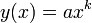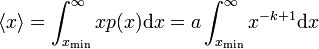# Power law

A power law is a mathematical relationship between two quantities where one is proportional to a power of the other: that is, of the form,$y(x) = ax^k\!$

where a and k are constants, with k being referred to as the exponent. Plotted on a log-log graph, this appears as a linear relationship with a slope of k, since$\log\left(y\right) = k \log x + \log a$

which has the same form, Y = mX + b, as a straight line. Equations that do not follow the above formula strictly may display power law tails, meaning that the ratio y(x) / axk tends towards one as as$x \to \infty$.

Strictly speaking the term "power law" includes many well-known formulas, such as those for calculating areas or volumes (e.g. πr2 for the area of a circle), Newton's inverse-square law of gravity, and so on. However, the term is typically used in the context of power-law probability distributions such as the Gutenberg-Richter law for earthquake sizes, or scaling relationships such as those observed in fractals, 1/f noise and allometric scaling laws in living organisms. Much of the interest springs from the great variety of natural situations in which such power laws are observed, and their occurrence as a common feature of diverse complex systems. Explanations for these findings remain a topic of considerable debate in the scientific literature.

## Properties of power laws

### Exponents, scale invariance and universality

One of the key properties of power laws is their scale invariance. Suppose that for a given power law, y(x) = axk, we change the length scale of our observation from x to Ax, where A is a constant. Then,$y(Ax) = a(Ax)^k = aA^{k}x^{k} = A^{k}ax^{k} = A^{k}y(x)\!$

which leaves the power law intact, changing only the constant of proportionality. It follows that power laws with the same exponent are to some extent equivalent, since each is simply a rescaling of the other.

In some cases this equivalence is reflected in the dynamical origins of power laws. For example, phase transitions in thermodynamic systems are associated with the emergence of power-law distributions of certain quantities, whose exponents are referred to as the critical exponents of the system. Diverse systems with the same critical exponents — that is, which display identical scaling behaviour as they approach criticality — can be shown, via renormalization group theory, to share the same fundamental dynamics. Similar observations have been made, though not as comprehensively, for various self-organized critical systems, where the critical point of the system is an attractor. Formally, this sharing of dynamics is referred to as universality, and systems with the same critical exponents are said to belong to the same universality class.

### Measuring the exponent from empirical data

Since a log-log plot of a power law yields a straight line, one simple way to estimate the exponent would be to perform linear regression on the log-values of the data. Unfortunately this method can produce wildly inaccurate estimates, as can be demonstrated by testing a randomly-generated data set from a known power law distribution.

An unbiased method, based on maximum likelihood estimation, chooses the maximally probable value for the exponent based on a given set of data points.

Given a set of real-valued data points {xi},$i = 1, \dots , N$,$k = 1 + N \left[ \sum_{i=1}^{N} \ln \frac{x_{i}}{x_{\mathrm{min}}} \right]^{-1}$

For a set of integer-valued data points {xi},$i = 1, \dots , N$, the maximum likelihood exponent is the solution to the transcendental equation$\frac{\zeta'(k)}{\zeta(k)} = -\frac{1}{N} \sum_{i=1}^{N} \ln x_{i}$

Note first that in this case, there is no value of xmin in the equation, so the power law is assumed to range from 1 to$\infty$. Further, these two equations are not equivalent, and the continuous version should not be applied to discrete data, nor vice versa.

## Power law probability distributions (Pareto distributions)

Power law probability distributions, frequently referred to as Pareto distributions in honour of the economist Vilfredo Pareto who introduced them in the late 19th century, describe many phenomena in nature, for example the Gutenberg-Richter law for the distribution of earthquake sizes. If we suppose a distribution to be of the form p(x) = axk, where x is a continuous variable, then aside from the above-mentioned scale invariance, a number of other features are observed.

To begin with, if we attempt to calculate the mean of x, we find,$\langle x \rangle = \int_{x_{\mathrm{min}}}^{\infty} x p(x) \mathrm{d}x = a \int_{x_{\mathrm{min}}}^{\infty} x^{-k+1} \mathrm{d}x$

In the special case k = 2 this is of course the integral of 1 / x, which yields,$\langle x \rangle = a [\log x]_{x_{\mathrm{min}}}^{\infty}$

while for k ≠ 2 we have,$\langle x \rangle = \frac{a}{2 - k}[x^{-k+2}]_{x_{\mathrm{min}}}^{\infty}$

It follows that the mean is finite only if k > 2, since for k ≤ 2 the above integral diverges.

If now we try instead to calculate the (complementary) cumulative distribution, P(x) = Pr(x' > x),$P(x) = \int_{x}^{\infty} p(x')\mathrm{d}x' = a\int_{x}^{\infty} x'^{-k} \mathrm{d}x'= \frac{a}{k-1} x^{-(k-1)}$

Thus, P(x) also follows a power law, with exponent (k – 1). This observation can be particularly useful when giving a graphical representation of a power law: whereas plotting p(x) accurately requires an appropriate choice of bin width for the data, P(x) is well defined for every value of x, and so avoids the possibility that a wrong choice of binning skews the value of k displayed on a graphical plot.